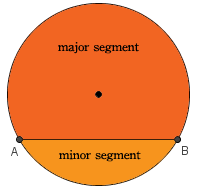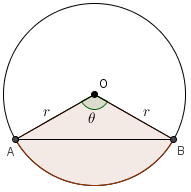# 弓形面積計算 Area of circular segments

• 17/07/2015\begin{align*} \text{Area of segment }&= \pi r^2 \cdot \frac{\theta}{360} – \frac{1}{2}(r)(r)\sin \theta\\[3pt] &= \pi r^2 \cdot \frac{\theta}{360} – \frac{1}{2}r^2\sin \theta \end{align*}

area of segment = area of sector OAB − area of OAB

• 2023/II/Q16
• 2022/II/Q16